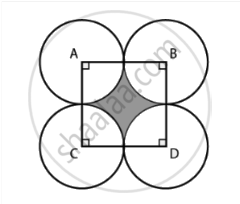# Four circular cardboard pieces of radii 7 cm are placed on a paper in such a way that each piece touches other two pieces. Find the area of the portion enclosed between these pieces. - Mathematics

Sum

Four circular cardboard pieces of radii 7 cm are placed on a paper in such a way that each piece touches other two pieces. Find the area of the portion enclosed between these pieces.

#### Solution

The four circles are placed such that each piece touches the other two piecesBy joining the centres of the circles by a line segment, we get a square ABDC with sides,

AB = BD = DC = CA = 2(Radius)

= 2(7) cm

= 14 cm

Area of the square = (Side)2

= (14)2

= 196 cm2

ABDC is a square

So, each angle has a measure of 90°.

∠A = ∠B = ∠D = ∠C = 90 = pi/2 radius

= θ   ....(Let)

Radius of each sector = 7 cm

Area of the sector with central angle A = (1/2) r^2 theta

= 11/2 r^2 theta

= 1/2 xx 49 xx pi/2

= 1/2 xx 49 xx 22/(2 xx 7)

= 77/2  cm^2

As the central angles and the radius of each sector are same, area of each sector is 77/2  cm^2.

Area of the shaded portion = Area of square – Area of the four sectors

= 196 - (4 xx 77/2)

= 196 - 154

= 42  cm^2

Therefore, required area of the portion enclosed between these pieces is 42 cm2.

Concept: Areas of Sector and Segment of a Circle
Is there an error in this question or solution?
Chapter 11: Area Related To Circles - Exercise 11.4 [Page 133]

#### APPEARS IN

NCERT Mathematics Exemplar Class 10
Chapter 11 Area Related To Circles
Exercise 11.4 | Q 9 | Page 133
Share